Comparison
Comparison close
Results

CCM.P-K2, EURAMET.M.P-K4.2010 and APMP.M.P-K9

MEASURAND                     Effective area of a piston-cylinder assembly

NOMINAL VALUE              335.7 mm2

NOMINAL PRESSURE        10 kPa

CCM.P-K2

Degrees of equivalence represented by Di = (xxR) and its expanded uncertainty Ui at a 95 % level of confidence, both expressed in mm2.

EURAMET.M.P-K4.2010 and APMP.M.P-K9

Degrees of equivalence represented by Di = (xI - xR) and its expanded uncertainty Ui at a 95 % level of confidence, both expressed in Pa.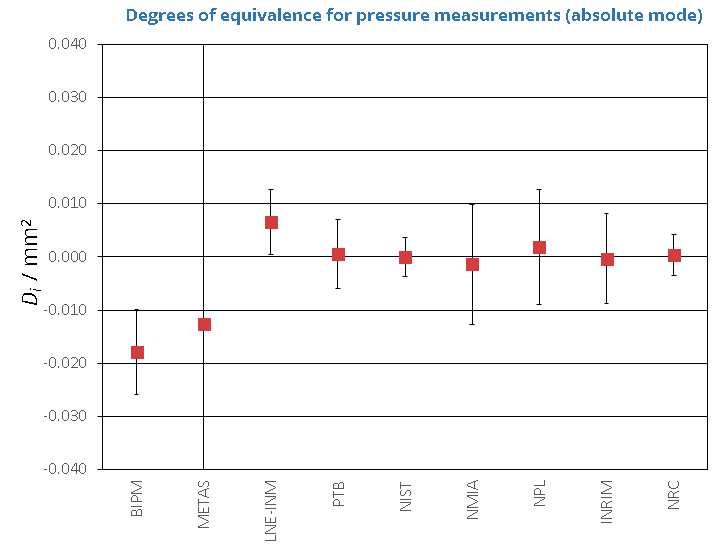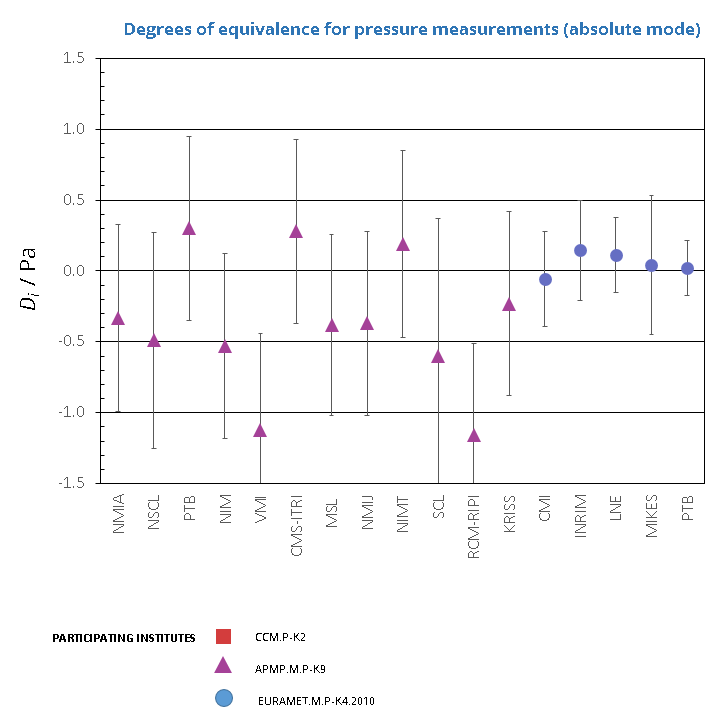CCM.P-K2 and EURAMET.M.P-K8

MEASURAND                     Effective area of a piston-cylinder assembly

NOMINAL VALUE              335.7 mm2

NOMINAL PRESSURE       20 kPa

Degrees of equivalence represented by Di = (xxR) and its expanded uncertainty Ui at a 95 % level of confidence, both expressed in mm2.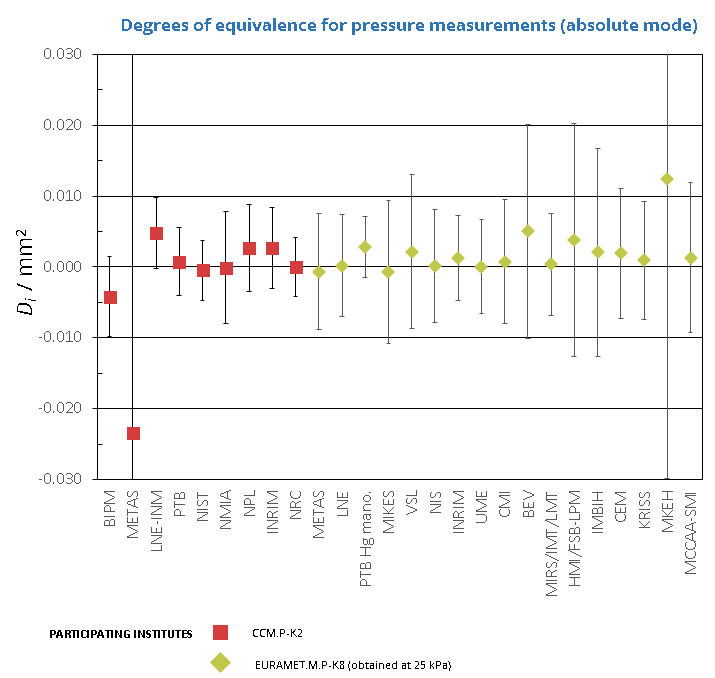CCM.P-K2 and APMP.M.P-K9

MEASURAND                     Effective area of a piston-cylinder assembly

NOMINAL VALUE              335.7 mm2

NOMINAL PRESSURE       30 kPa

CCM.P-K2

Degrees of equivalence represented by Di = (xxR) and its expanded uncertainty Ui at a 95 % level of confidence, both expressed in mm2.

APMP.M.P-K9

Degrees of equivalence represented by Di = (xI - xR) and its expanded uncertainty Ui at a 95 % level of confidence, both expressed in Pa.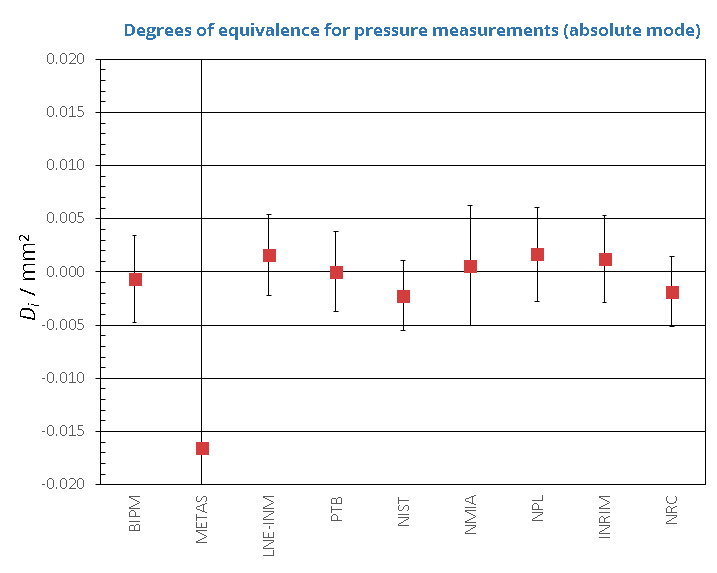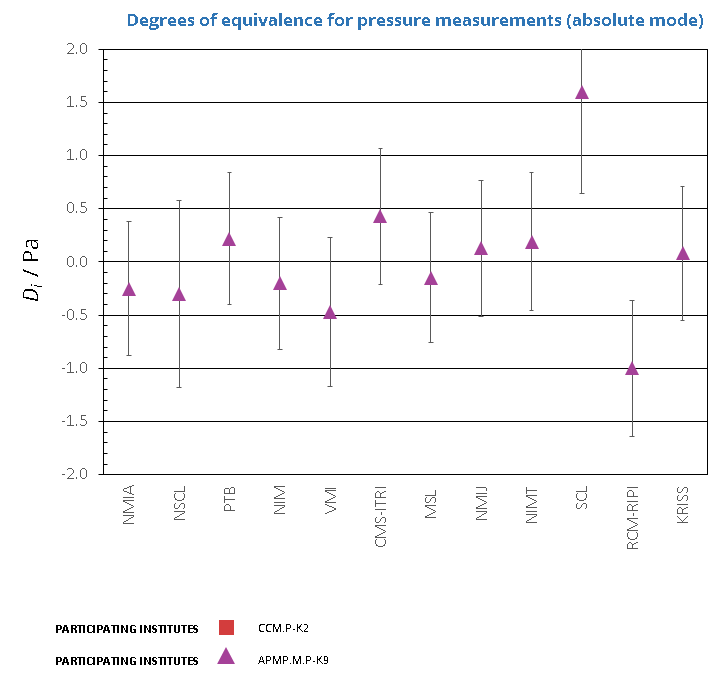CCM.P-K2

MEASURAND                     Effective area of a piston-cylinder assembly

NOMINAL VALUE              335.7 mm2

NOMINAL PRESSURE       40 kPa

Degrees of equivalence represented by Di = (xxR) and its expanded uncertainty Ui at a 95 % level of confidence, both expressed in mm2.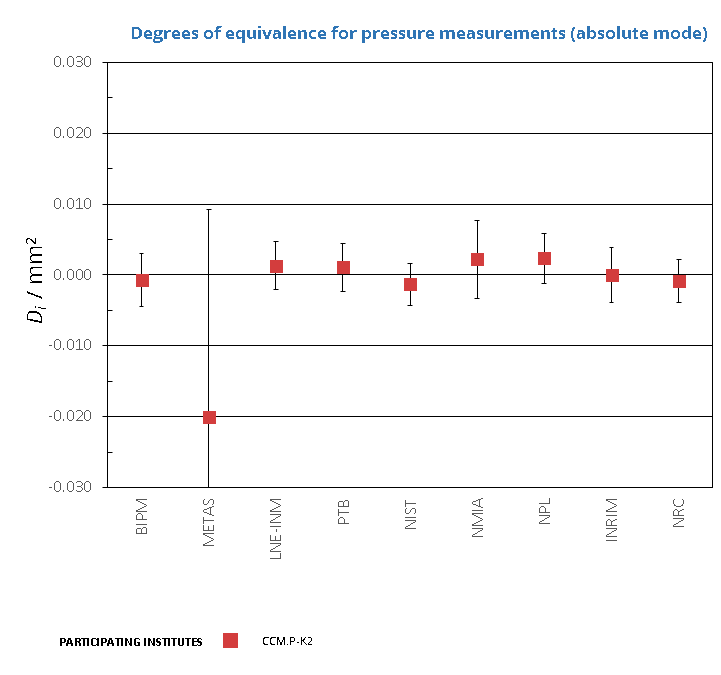CCM.P-K2, EURAMET.M.P-K8 and APMP.M.P-K9

MEASURAND                     Effective area of a piston-cylinder assembly

NOMINAL VALUE              335.7 mm2

NOMINAL PRESSURE       50 kPa

CCM.P-K2 and EURAMET.M.P-K8

Degrees of equivalence represented by Di = (xxR) and its expanded uncertainty Ui at a 95 % level of confidence, both expressed in mm2.

APMP.M.P-K9

Degrees of equivalence represented by Di = (xI - xR) and its expanded uncertainty Ui at a 95 % level of confidence, both expressed in Pa.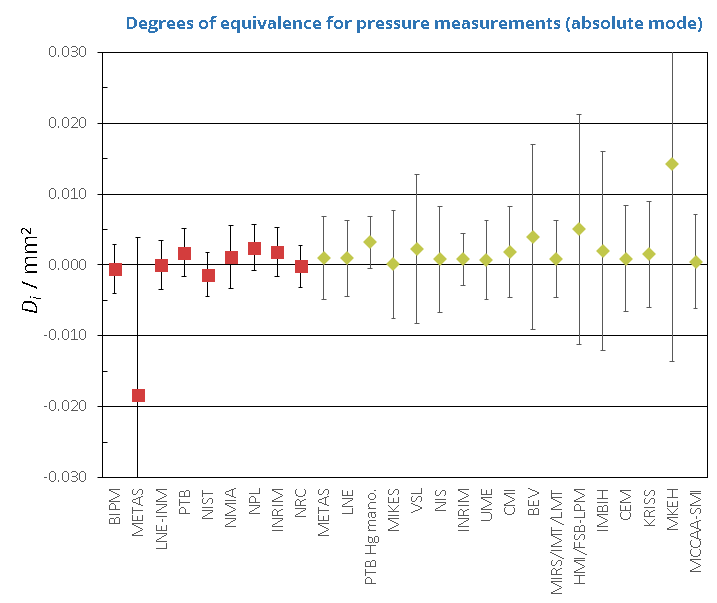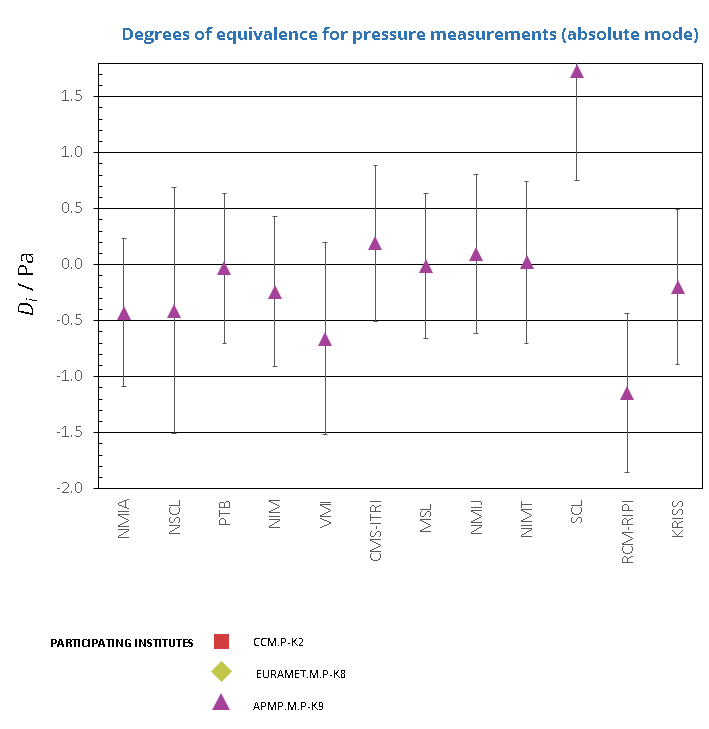CCM.P-K2

MEASURAND                     Effective area of a piston-cylinder assembly

NOMINAL VALUE              335.7 mm2

NOMINAL PRESSURE       60 kPa

Degrees of equivalence represented by Di = (xxR) and its expanded uncertainty Ui at a 95 % level of confidence, both expressed in mm2.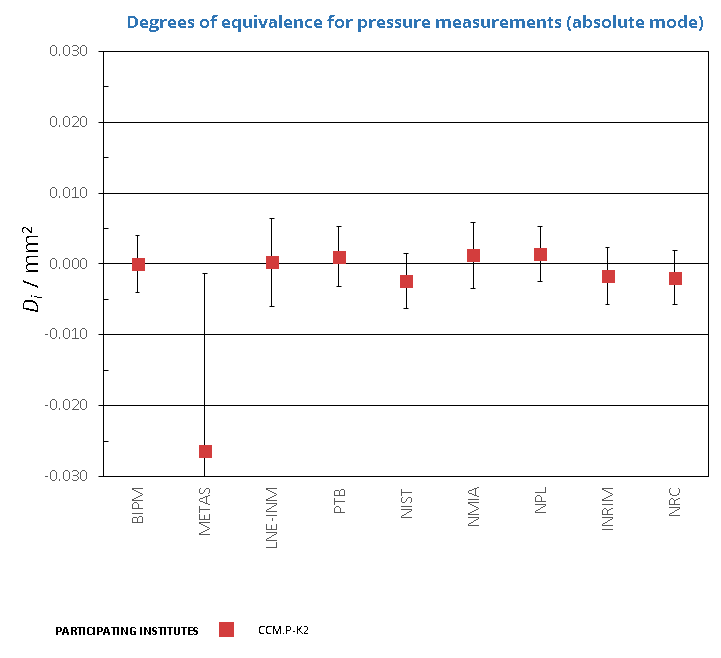CCM.P-K2, EURAMET.M.P-K8 and APMP.M.P-K9

MEASURAND                     Effective area of a piston-cylinder assembly

NOMINAL VALUE              335.7 mm2

NOMINAL PRESSURE       70 kPa

CCM.P-K2 and EURAMET.M.P-K8

Degrees of equivalence represented by Di = (xxR) and its expanded uncertainty Ui at a 95 % level of confidence, both expressed in mm2.

APMP.M.P-K9

Degrees of equivalence represented by Di = (xI - xR) and its expanded uncertainty Ui at a 95 % level of confidence, both expressed in Pa.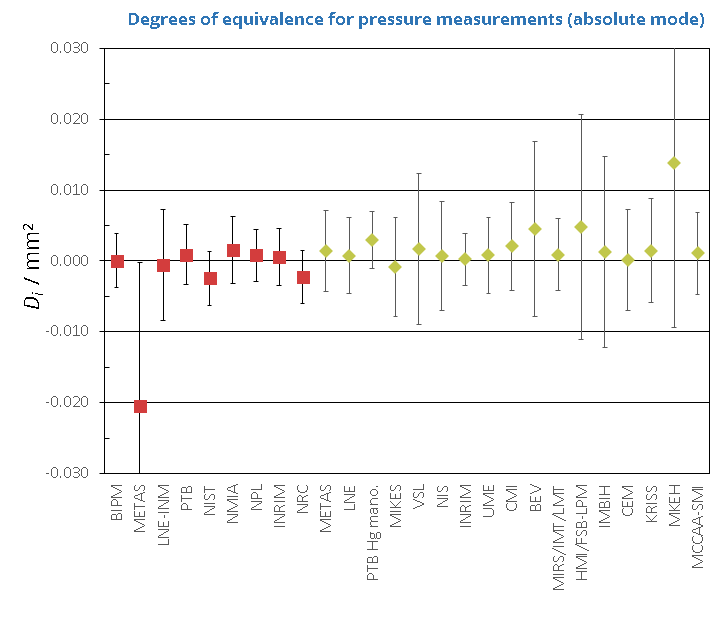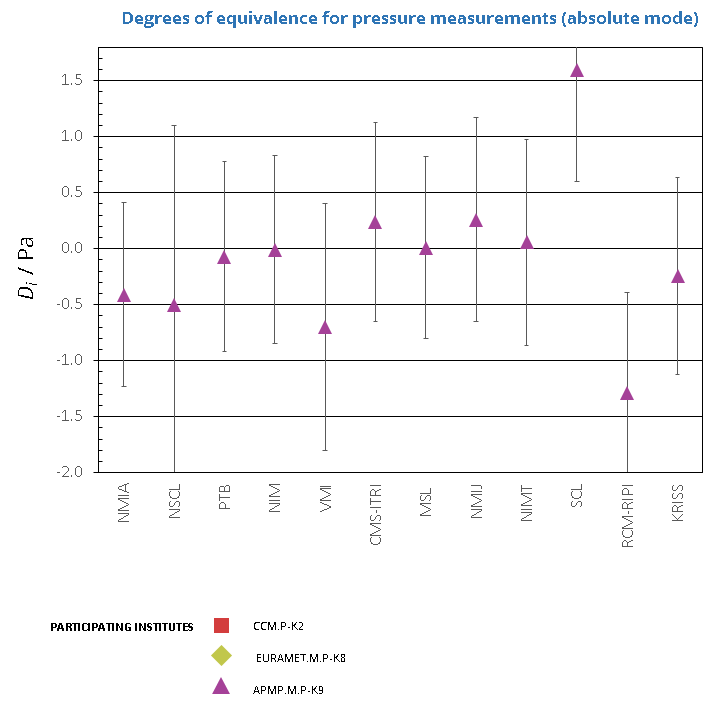CCM.P-K2

MEASURAND                     Effective area of a piston-cylinder assembly

NOMINAL VALUE              335.7 mm2

NOMINAL PRESSURE       80 kPa

Degrees of equivalence represented by Di = (xxR) and its expanded uncertainty Ui at a 95 % level of confidence, both expressed in mm2.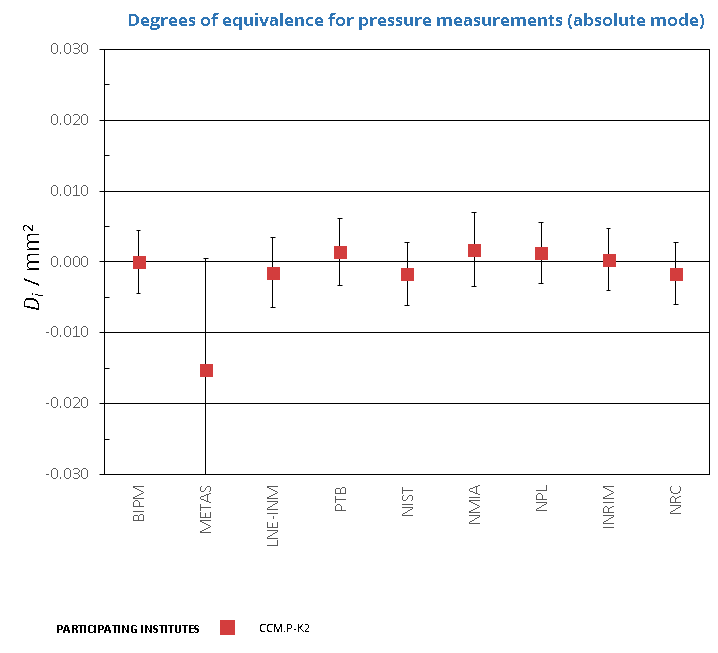CCM.P-K2 and APMP.M.P-K9

MEASURAND                     Effective area of a piston-cylinder assembly

NOMINAL VALUE              335.7 mm2

NOMINAL PRESSURE       90 kPa

CCM.P-K2

Degrees of equivalence represented by Di = (xxR) and its expanded uncertainty Ui at a 95 % level of confidence, both expressed in mm2.

APMP.M.P-K9

Degrees of equivalence represented by Di = (xI - xR) and its expanded uncertainty Ui at a 95 % level of confidence, both expressed in Pa.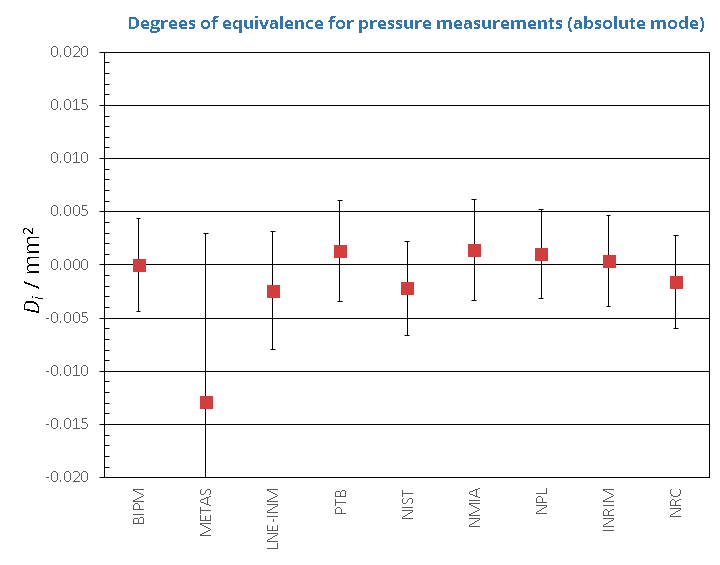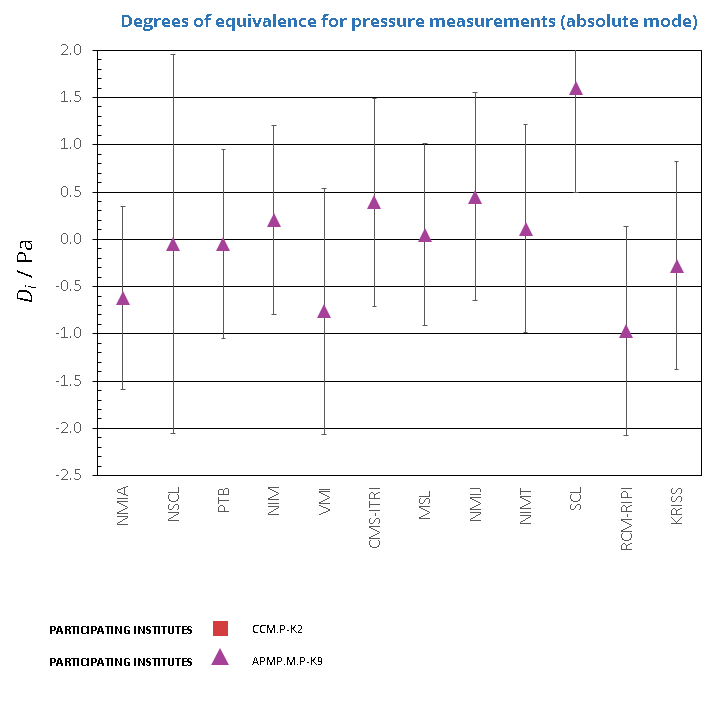CCM.P-K2, EURAMET.M.P-K8 and APMP.M.P-K9

MEASURAND                     Effective area of a piston-cylinder assembly

NOMINAL VALUE              335.7 mm2

NOMINAL PRESSURE       100 kPa

CCM.P-K2 and EURAMET.M.P-K8

Degrees of equivalence represented by Di = (xxR) and its expanded uncertainty Ui at a 95 % level of confidence, both expressed in mm2.

APMP.M.P-K9

Degrees of equivalence represented by Di = (xI - xR) and its expanded uncertainty Ui at a 95 % level of confidence, both expressed in Pa.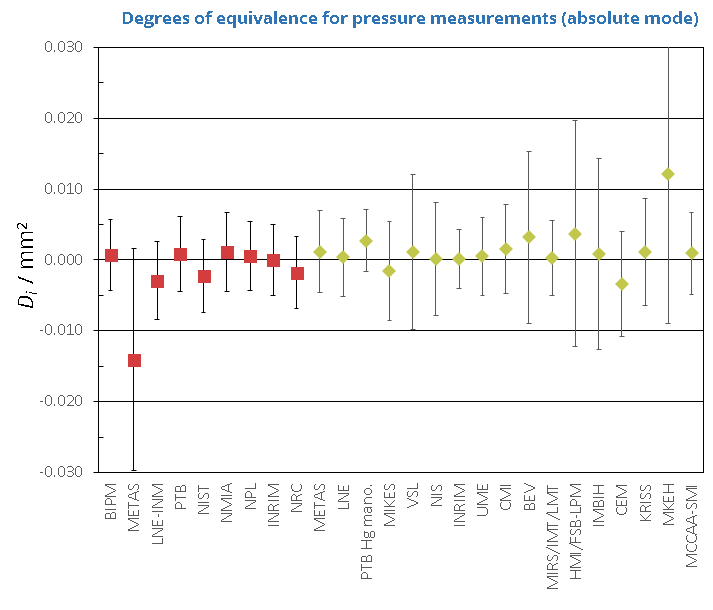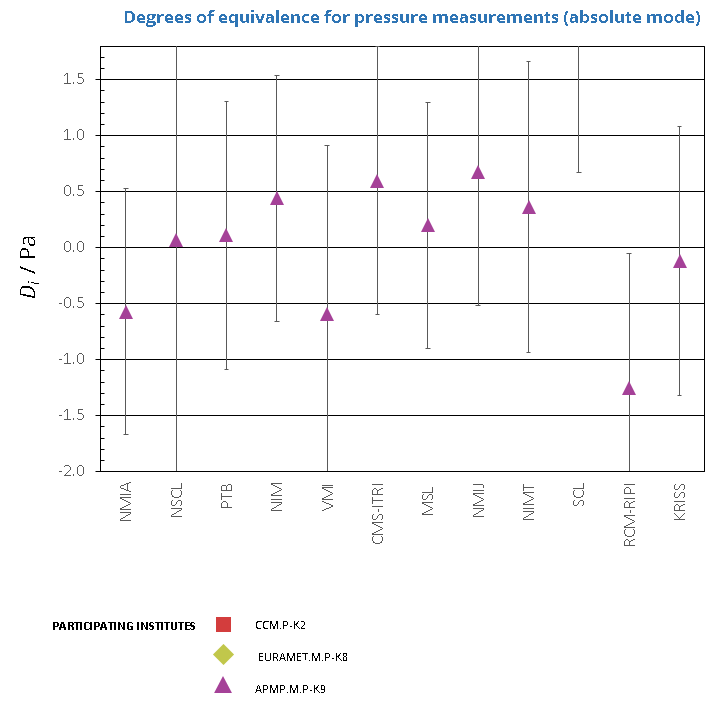CCM.P-K2 and APMP.M.P-K9

MEASURAND                     Effective area of a piston-cylinder assembly

NOMINAL VALUE              335.7 mm2

NOMINAL PRESSURE       110 kPa

CCM.P-K2

Degrees of equivalence represented by Di = (xxR) and its expanded uncertainty Ui at a 95 % level of confidence, both expressed in mm2.

APMP.M.P-K9

Degrees of equivalence represented by Di = (xI - xR) and its expanded uncertainty Ui at a 95 % level of confidence, both expressed in Pa.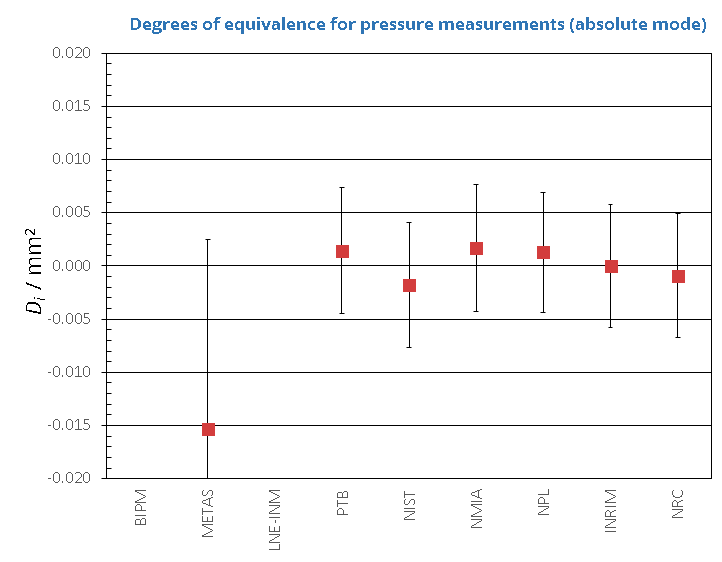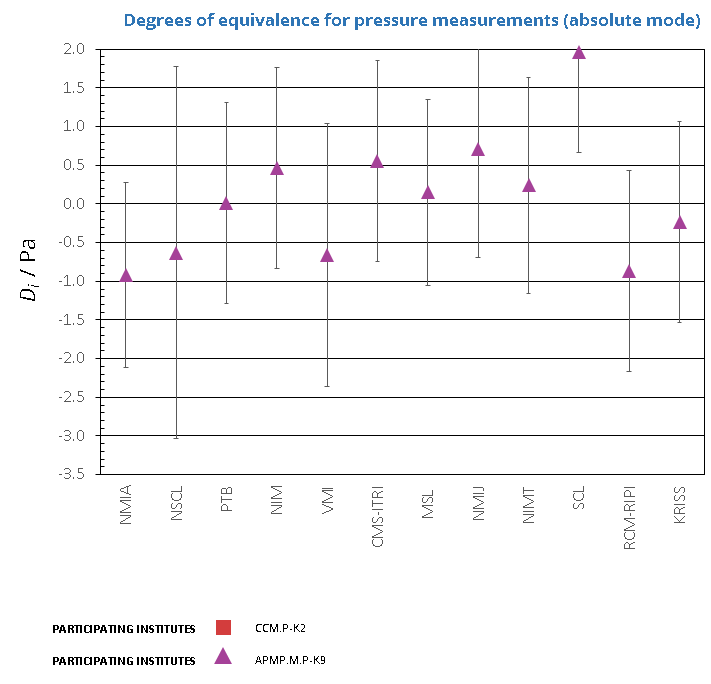CCM.P-K2

MEASURAND                     Effective area of a piston-cylinder assembly

NOMINAL VALUE              335.7 mm2

NOMINAL PRESSURE       120 kPa

Degrees of equivalence represented by Di = (xxR) and its expanded uncertainty Ui at a 95 % level of confidence, both expressed in mm2.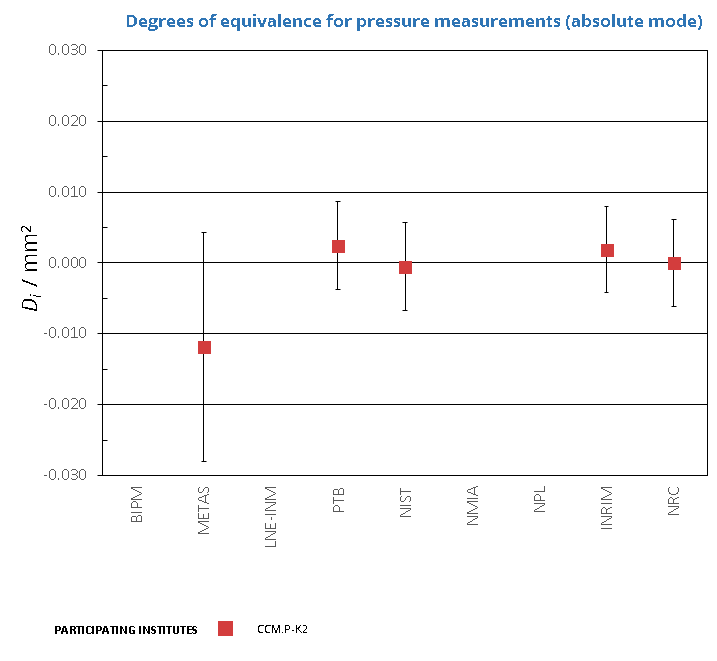Comparison
Comparison close
Results

CCM.P-K2, EURAMET.M.P-K4.2010 and APMP.M.P-K9

MEASURAND                     Effective area of a piston-cylinder assembly

NOMINAL VALUE              335.7 mm2

NOMINAL PRESSURE        10 kPa

CCM.P-K2

Degrees of equivalence represented by Di = (xxR) and its expanded uncertainty Ui at a 95 % level of confidence, both expressed in mm2.

EURAMET.M.P-K4.2010 and APMP.M.P-K9

Degrees of equivalence represented by Di = (xI - xR) and its expanded uncertainty Ui at a 95 % level of confidence, both expressed in Pa.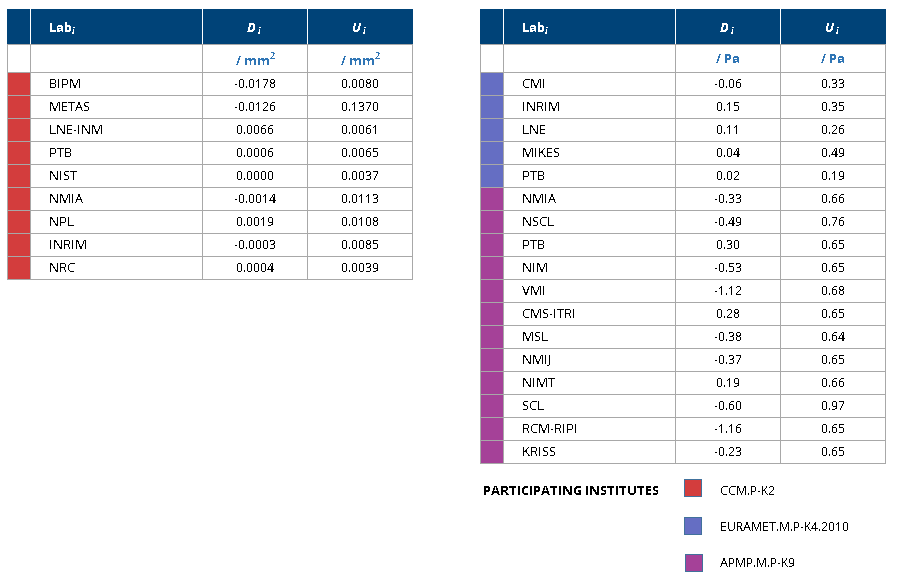CCM.P-K2 and EURAMET.M.P-K8

MEASURAND                     Effective area of a piston-cylinder assembly

NOMINAL VALUE              335.7 mm2

NOMINAL PRESSURE       20 kPa

Degrees of equivalence represented by Di = (xxR) and its expanded uncertainty Ui at a 95 % level of confidence, both expressed in mm2.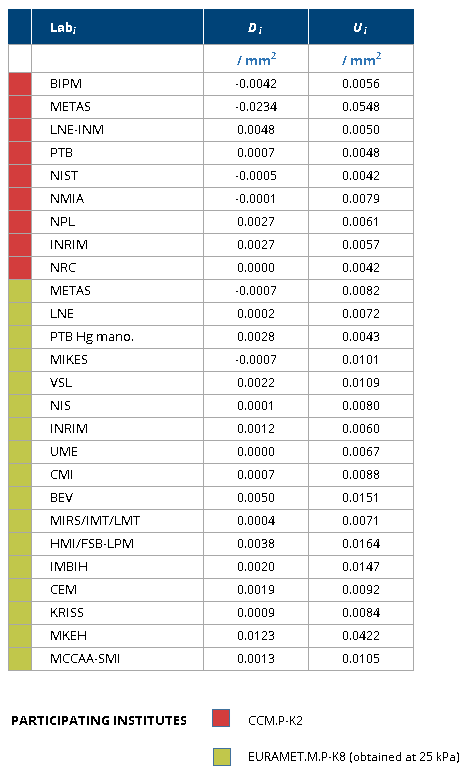CCM.P-K2 and APMP.M.P-K9

MEASURAND                     Effective area of a piston-cylinder assembly

NOMINAL VALUE              335.7 mm2

NOMINAL PRESSURE       30 kPa

CCM.P-K2

Degrees of equivalence represented by Di = (xxR) and its expanded uncertainty Ui at a 95 % level of confidence, both expressed in mm2.

APMP.M.P-K9

Degrees of equivalence represented by Di = (xI - xR) and its expanded uncertainty Ui at a 95 % level of confidence, both expressed in Pa.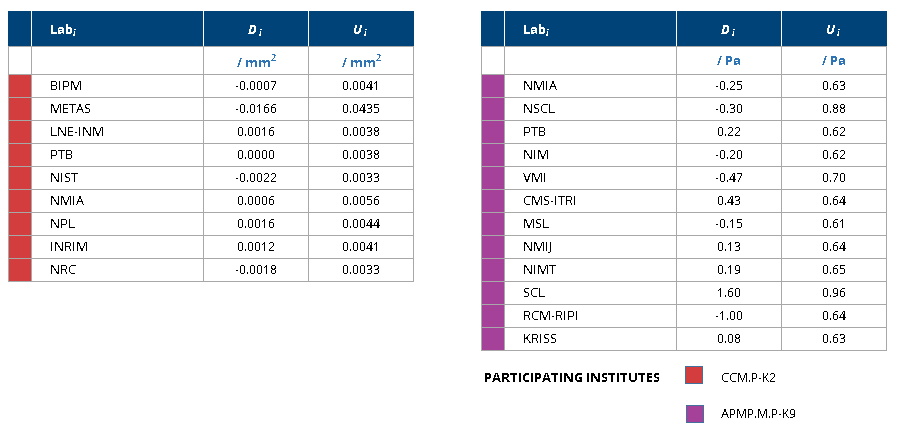CCM.P-K2

MEASURAND                     Effective area of a piston-cylinder assembly

NOMINAL VALUE              335.7 mm2

NOMINAL PRESSURE       40 kPa

Degrees of equivalence represented by Di = (xxR) and its expanded uncertainty Ui at a 95 % level of confidence, both expressed in mm2.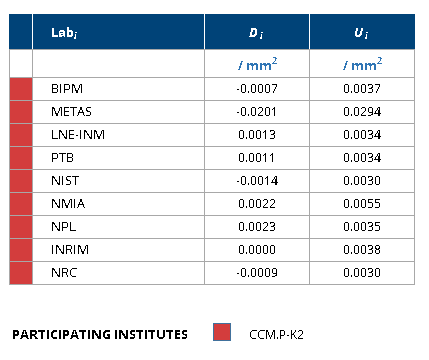CCM.P-K2, EURAMET.M.P-K8 and APMP.M.P-K9

MEASURAND                     Effective area of a piston-cylinder assembly

NOMINAL VALUE              335.7 mm2

NOMINAL PRESSURE       50 kPa

CCM.P-K2 and EURAMET.M.P-K8

Degrees of equivalence represented by Di = (xxR) and its expanded uncertainty Ui at a 95 % level of confidence, both expressed in mm2.

APMP.M.P-K9

Degrees of equivalence represented by Di = (xI - xR) and its expanded uncertainty Ui at a 95 % level of confidence, both expressed in Pa.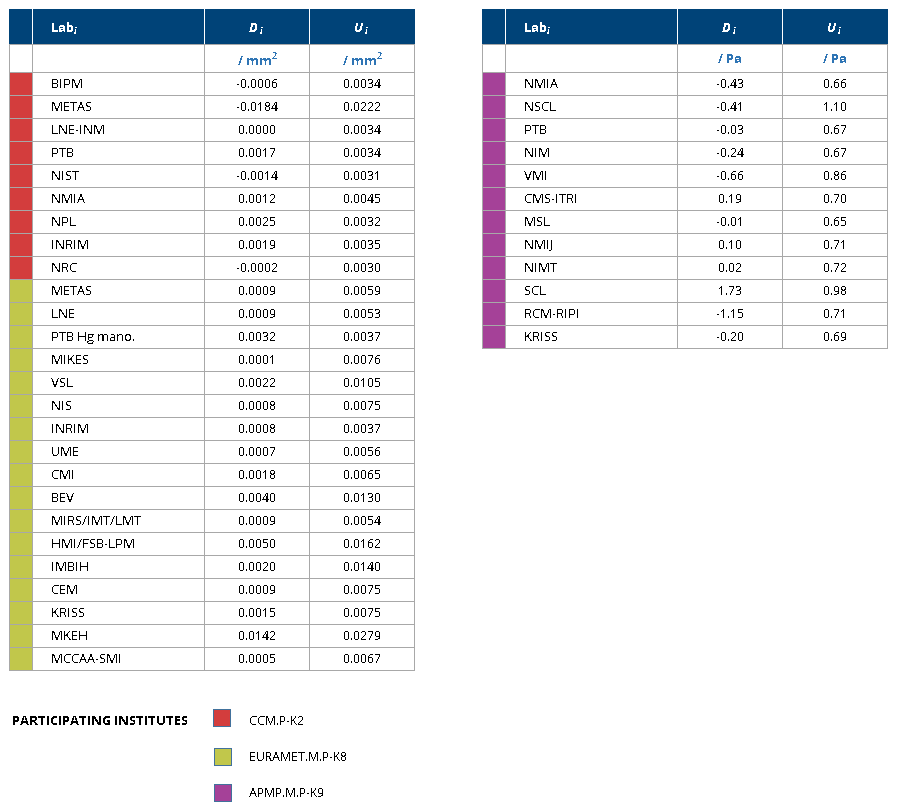CCM.P-K2

MEASURAND                     Effective area of a piston-cylinder assembly

NOMINAL VALUE              335.7 mm2

NOMINAL PRESSURE       60 kPa

Degrees of equivalence represented by Di = (xxR) and its expanded uncertainty Ui at a 95 % level of confidence, both expressed in mm2.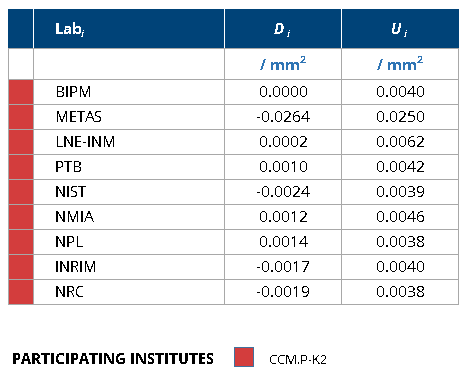CCM.P-K2, EURAMET.M.P-K8 and APMP.M.P-K9

MEASURAND                     Effective area of a piston-cylinder assembly

NOMINAL VALUE              335.7 mm2

NOMINAL PRESSURE       70 kPa

CCM.P-K2 and EURAMET.M.P-K8

Degrees of equivalence represented by Di = (xxR) and its expanded uncertainty Ui at a 95 % level of confidence, both expressed in mm2.

APMP.M.P-K9

Degrees of equivalence represented by Di = (xI - xR) and its expanded uncertainty Ui at a 95 % level of confidence, both expressed in Pa.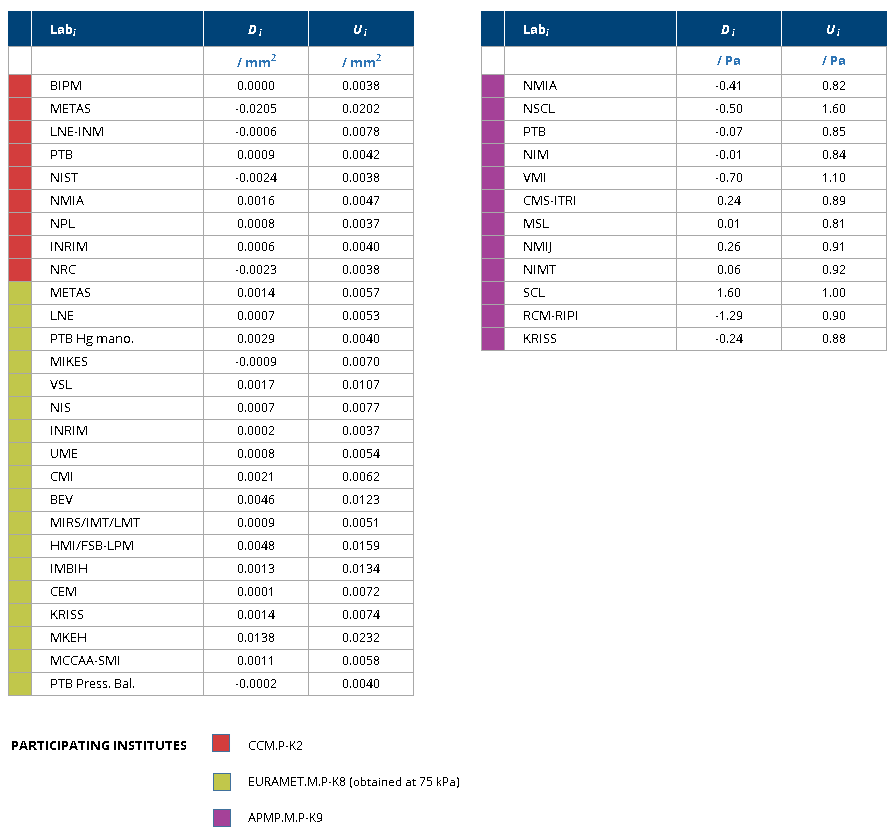CCM.P-K2

MEASURAND                     Effective area of a piston-cylinder assembly

NOMINAL VALUE              335.7 mm2

NOMINAL PRESSURE       80 kPa

Degrees of equivalence represented by Di = (xxR) and its expanded uncertainty Ui at a 95 % level of confidence, both expressed in mm2.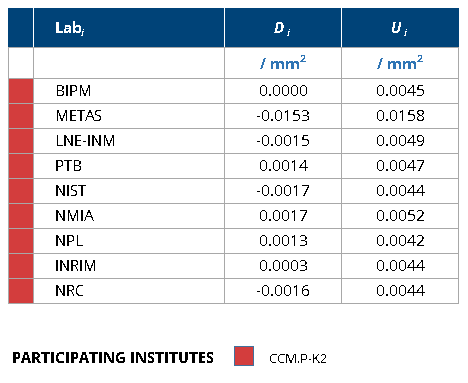CCM.P-K2 and APMP.M.P-K9

MEASURAND                     Effective area of a piston-cylinder assembly

NOMINAL VALUE              335.7 mm2

NOMINAL PRESSURE       90 kPa

CCM.P-K2

Degrees of equivalence represented by Di = (xxR) and its expanded uncertainty Ui at a 95 % level of confidence, both expressed in mm2.

APMP.M.P-K9

Degrees of equivalence represented by Di = (xI - xR) and its expanded uncertainty Ui at a 95 % level of confidence, both expressed in Pa.CCM.P-K2, EURAMET.M.P-K8 and APMP.M.P-K9

MEASURAND                     Effective area of a piston-cylinder assembly

NOMINAL VALUE              335.7 mm2

NOMINAL PRESSURE       100 kPa

CCM.P-K2 and EURAMET.M.P-K8

Degrees of equivalence represented by Di = (xxR) and its expanded uncertainty Ui at a 95 % level of confidence, both expressed in mm2.

APMP.M.P-K9

Degrees of equivalence represented by Di = (xI - xR) and its expanded uncertainty Ui at a 95 % level of confidence, both expressed in Pa.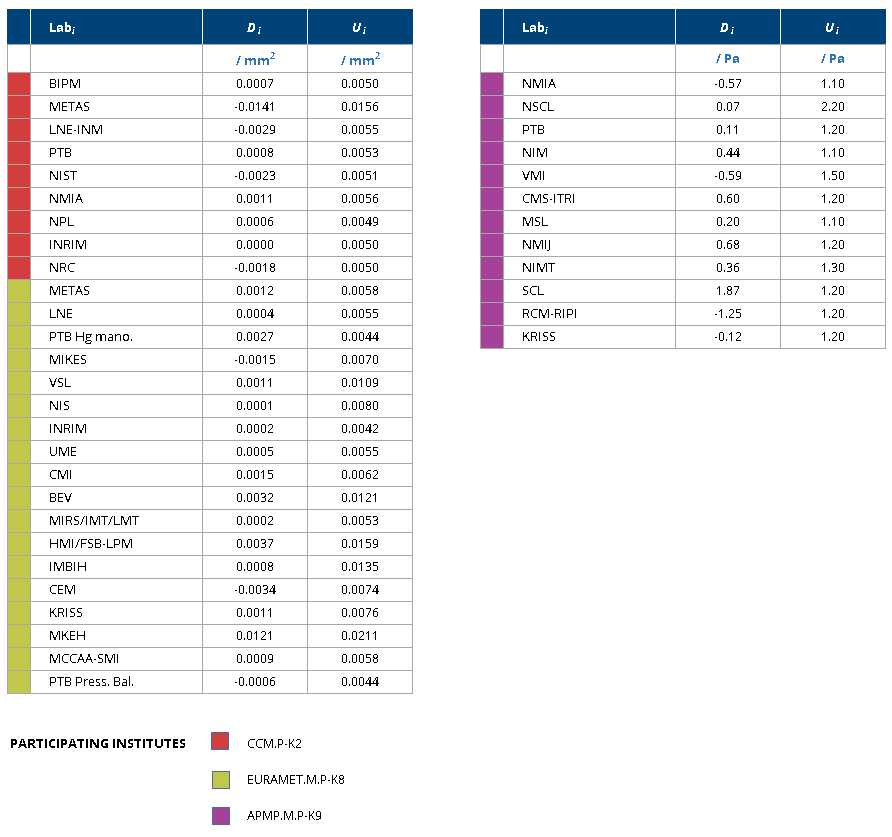CCM.P-K2 and APMP.M.P-K9

MEASURAND                     Effective area of a piston-cylinder assembly

NOMINAL VALUE              335.7 mm2

NOMINAL PRESSURE       110 kPa

CCM.P-K2

Degrees of equivalence represented by Di = (xxR) and its expanded uncertainty Ui at a 95 % level of confidence, both expressed in mm2.

APMP.M.P-K9

Degrees of equivalence represented by Di = (xI - xR) and its expanded uncertainty Ui at a 95 % level of confidence, both expressed in Pa.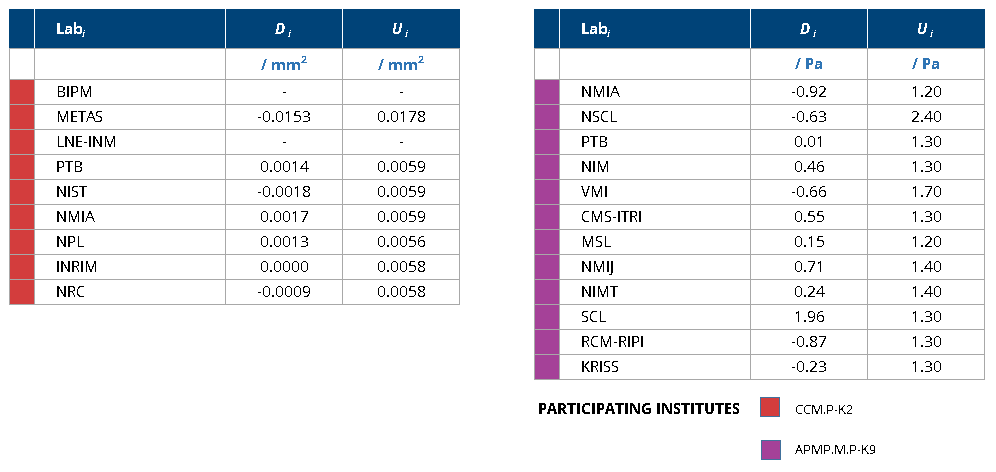CCM.P-K2

MEASURAND                     Effective area of a piston-cylinder assembly

NOMINAL VALUE              335.7 mm2

NOMINAL PRESSURE       120 kPa

Degrees of equivalence represented by Di = (xxR) and its expanded uncertainty Ui at a 95 % level of confidence, both expressed in mm2.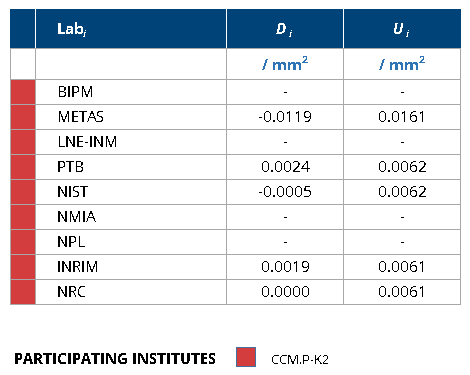Comparison
Comparison close
CC comparison
 Metrology area, Sub-field Mass and related quantities, Pressure Description Pressure measurements (absolute mode) Time of measurements 2009 - 2013 Status Approved for equivalence
 Final Reports of the comparisons Measurand Pressure 10 kPa to 110 kPa (absolute mode) Transfer device Precise digital pressure transfer standard
 Comparison type Key Comparison Consultative Committee CCM (Consultative Committee for Mass and Related Quantities) Conducted by APMP (Asia Pacific Metrology Programme) Comments Results published on 3 June 2020 Pilot institute KRISS Korea Research Institute of Standards and Science Korea, Republic of Contact person Sam-Yong Woo +82 42 868 5118 Additional
First Name Last Name
wwww@ww.www +356719836 Institute 1 Institute 1 Khmelnitskiy
Pilot laboratory
KRISS

Korea Research Institute of Standards and Science, Korea, Republic of, APMP

 MSL Measurement Standards Laboratory, New Zealand, APMP
 NIM National Institute of Metrology, China, APMP
 NIMT National Institute of Metrology (Thailand), Thailand, APMP
 NMIA National Measurement Institute, Australia, Australia, APMP
 NMIJ AIST National Metrology Institute of Japan, Japan, APMP
 NMIM National Metrology Institute of Malaysia, Malaysia, APMP
 NPLI CSIR National Physical Laboratory of India, India, APMP
 NSCL National Standards and Calibration Laboratory, Syrian Arab Republic, APMP
 PTB Physikalisch-Technische Bundesanstalt, Germany, EURAMET
 RCM-LIPI Research Center for Metrology-LIPI, Indonesia, APMP
 SCL Standards and Calibration Laboratory, Hong Kong, China, APMP
Comparison
Comparison close
Results

CCM.P-K2, EURAMET.M.P-K4.2010 and APMP.M.P-K9

MEASURAND                     Effective area of a piston-cylinder assembly

NOMINAL VALUE              335.7 mm2

NOMINAL PRESSURE        10 kPa

CCM.P-K2

The key comparison reference value, xR, is calculated as the median of the participants' values. The standard uncertainty of the key comparison reference value, uR, is calculated by the formula uR = 1.858 MAD / (n - 1)1/2, where MAD is the median of absolute deviations from the median, and n is the number of participants contributing to the reference value.

xR = 335.744 4 mm2
uR = 0.000 9 mm2

The degree of equivalence of laboratory i with respect to the key comparison reference value is given by a pair of terms expressed in mm2:

Di = (xi - xR) and Ui = 2(ui2 + uR2 + uT2)1/2, its expanded uncertainty (k = 2). uT is the uncertainty due to the instability of the transfer standard: uT = 0.002 5 mm2 (see page 15 of the Final Report).

The degree of equivalence between two laboratories i and j is given by a pair of terms expressed in mm2Dij = Di - Dj = (xi - xj) and Uij = 2(ui2 + uj2 + 2uT2)1/2, its expanded uncertainty (k = 2). Effects of correlation should be taken into consideration.

PTB provides the link between key comparisons CCM.P-K2 and EURAMET.M.P-K4.2010, having participated in both comparisons. The linkage process is described in Section 11 of the EURAMET.M.P-K4.2010 Final Report.

The degrees of equivalence of the laboratories having participated in EURAMET.M.P-K4.2010, with respect to the CCM.P-K2 key comparison reference value, are expressed in Pa, and therefore are shown on a different graph from the one for the participants in CCM.P-K2.

NMIA and PTB provides the link between key comparisons CCM.P-K2 and APMP.M.P-K9, having participated in both comparisons. The linkage process is described in Section 6 of the APMP.M.P-K9 Final Report.

The degrees of equivalence of the laboratories having participated in APMP.M.P-K9, with respect to the CCM.P-K2 key comparison reference value, are expressed in Pa, and therefore are shown on a different graph from the one for the participants in CCM.P-K2.

CCM.P-K2 and EURAMET.M.P-K8

MEASURAND                     Effective area of a piston-cylinder assembly

NOMINAL VALUE              335.7 mm2

NOMINAL PRESSURE       20 kPa

CCM.P-K2

The key comparison reference value, xR, is calculated as the median of the participants' values. The standard uncertainty of the key comparison reference value, uR, is calculated by the formula uR = 1.858 MAD / (n - 1)1/2, where MAD is the median of absolute deviations from the median, and n is the number of participants contributing to the reference value.

xR = 335.744 8 mm2
uR = 0.001 7 mm2

The degree of equivalence of laboratory i with respect to the key comparison reference value is given by a pair of terms expressed in mm2:

Di = (xi - xR) and Ui = 2(ui2 + uR2 + uT2)1/2, its expanded uncertainty (k = 2). uT is the uncertainty due to the instability of the transfer standard: uT = 0.002 5 mm2 (see page 15 of the Final Report).

The degree of equivalence between two laboratories i and j is given by a pair of terms expressed in mm2Dij = Di - Dj = (xi - xj) and Uij = 2(ui2 + uj2 + 2uT2)1/2, its expanded uncertainty (k = 2). Effects of correlation should be taken into consideration.

PTB and INRiM provides the link between key comparisons CCM.P-K2 and EURAMET.M.P-K8, having participated in both comparisons. The linkage process is described in Section 11 of the EURAMET.M.P-K8 Final Report.

The degrees of equivalence of the laboratories having participated in EURAMET.M.P-K4.2010, with respect to the CCM.P-K2 key comparison reference value, are expressed in Pa, and therefore are shown on a different graph from the one for the participants in CCM.P-K2.

CCM.P-K2 and APMP.M.P-K9

MEASURAND                     Effective area of a piston-cylinder assembly

NOMINAL VALUE              335.7 mm2

NOMINAL PRESSURE       30 kPa

CCM.P-K2

The key comparison reference value, xR, is calculated as the median of the participants' values. The standard uncertainty of the key comparison reference value, uR, is calculated by the formula uR = 1.858 MAD / (n - 1)1/2, where MAD is the median of absolute deviations from the median, and n is the number of participants contributing to the reference value.

xR = 335.745 5 mm2
uR = 0.001 0 mm2

The degree of equivalence of laboratory i with respect to the key comparison reference value is given by a pair of terms expressed in mm2:

Di = (xi - xR) and Ui = 2(ui2 + uR2 + uT2)1/2, its expanded uncertainty (k = 2). uT is the uncertainty due to the instability of the transfer standard: uT = 0.002 5 mm2 (see page 15 of the Final Report).

The degree of equivalence between two laboratories i and j is given by a pair of terms expressed in mm2Dij = Di - Dj = (xi - xj) and Uij = 2(ui2 + uj2 + 2uT2)1/2, its expanded uncertainty (k = 2). Effects of correlation should be taken into consideration.

NMIA and PTB provides the link between key comparisons CCM.P-K2 and APMP.M.P-K9, having participated in both comparisons. The linkage process is described in Section 6 of the APMP.M.P-K9 Final Report.

The degrees of equivalence of the laboratories having participated in APMP.M.P-K9, with respect to the CCM.P-K2 key comparison reference value, are expressed in Pa, and therefore are shown on a different graph from the one for the participants in CCM.P-K2.

CCM.P-K2

MEASURAND                     Effective area of a piston-cylinder assembly

NOMINAL VALUE              335.7 mm2

NOMINAL PRESSURE       40 kPa

The key comparison reference value, xR, is calculated as the median of the participants' values. The standard uncertainty of the key comparison reference value, uR, is calculated by the formula uR = 1.858 MAD / (n - 1)1/2, where MAD is the median of absolute deviations from the median, and n is the number of participants contributing to the reference value.

xR = 335.744 2 mm2
uR = 0.000 8 mm2

The degree of equivalence of laboratory i with respect to the key comparison reference value is given by a pair of terms expressed in mm2:

Di = (xi - xR) and Ui = 2(ui2 + uR2 + uT2)1/2, its expanded uncertainty (k = 2). uT is the uncertainty due to the instability of the transfer standard: uT = 0.002 5 mm2 (see page 15 of the Final Report).

The degree of equivalence between two laboratories i and j is given by a pair of terms expressed in mm2Dij = Di - Dj = (xi - xj) and Uij = 2(ui2 + uj2 + 2uT2)1/2, its expanded uncertainty (k = 2). Effects of correlation should be taken into consideration.

CCM.P-K2, EURAMET.M.P-K8 and APMP.M.P-K9

MEASURAND                     Effective area of a piston-cylinder assembly

NOMINAL VALUE              335.7 mm2

NOMINAL PRESSURE       50 kPa

CCM.P-K2

The key comparison reference value, xR, is calculated as the median of the participants' values. The standard uncertainty of the key comparison reference value, uR, is calculated by the formula uR = 1.858 MAD / (n - 1)1/2, where MAD is the median of absolute deviations from the median, and n is the number of participants contributing to the reference value.

xR = 335.744 0 mm2
uR = 0.000 8 mm2

The degree of equivalence of laboratory i with respect to the key comparison reference value is given by a pair of terms expressed in mm2:

Di = (xi - xR) and Ui = 2(ui2 + uR2 + uT2)1/2, its expanded uncertainty (k = 2). uT is the uncertainty due to the instability of the transfer standard: uT = 0.002 5 mm2 (see page 15 of the Final Report).

The degree of equivalence between two laboratories i and j is given by a pair of terms expressed in mm2Dij = Di - Dj = (xi - xj) and Uij = 2(ui2 + uj2 + 2uT2)1/2, its expanded uncertainty (k = 2). Effects of correlation should be taken into consideration.

PTB and INRiM provides the link between key comparisons CCM.P-K2 and EURAMET.M.P-K8, having participated in both comparisons. The linkage process is described in Section 11 of the EURAMET.M.P-K8 Final Report.

The degrees of equivalence of the laboratories having participated in EURAMET.M.P-K4.2010, with respect to the CCM.P-K2 key comparison reference value, are expressed in Pa, and therefore are shown on a different graph from the one for the participants in CCM.P-K2.

NMIA and PTB provides the link between key comparisons CCM.P-K2 and APMP.M.P-K9, having participated in both comparisons. The linkage process is described in Section 6 of the APMP.M.P-K9 Final Report.

The degrees of equivalence of the laboratories having participated in APMP.M.P-K9, with respect to the CCM.P-K2 key comparison reference value, are expressed in Pa, and therefore are shown on a different graph from the one for the participants in CCM.P-K2.

CCM.P-K2

MEASURAND                     Effective area of a piston-cylinder assembly

NOMINAL VALUE              335.7 mm2

NOMINAL PRESSURE       60 kPa

The key comparison reference value, xR, is calculated as the median of the participants' values. The standard uncertainty of the key comparison reference value, uR, is calculated by the formula uR = 1.858 MAD / (n - 1)1/2, where MAD is the median of absolute deviations from the median, and n is the number of participants contributing to the reference value.

xR = 335.745 1 mm2
uR = 0.000 9 mm2

The degree of equivalence of laboratory i with respect to the key comparison reference value is given by a pair of terms expressed in mm2:

Di = (xi - xR) and Ui = 2(ui2 + uR2 + uT2)1/2, its expanded uncertainty (k = 2). uT is the uncertainty due to the instability of the transfer standard: uT = 0.002 5 mm2 (see page 15 of the Final Report).

The degree of equivalence between two laboratories i and j is given by a pair of terms expressed in mm2Dij = Di - Dj = (xi - xj) and Uij = 2(ui2 + uj2 + 2uT2)1/2, its expanded uncertainty (k = 2). Effects of correlation should be taken into consideration.

CCM.P-K2, EURAMET.M.P-K8 and APMP.M.P-K9

MEASURAND                     Effective area of a piston-cylinder assembly

NOMINAL VALUE              335.7 mm2

NOMINAL PRESSURE       70 kPa

CCM.P-K2

The key comparison reference value, xR, is calculated as the median of the participants' values. The standard uncertainty of the key comparison reference value, uR, is calculated by the formula uR = 1.858 MAD / (n - 1)1/2, where MAD is the median of absolute deviations from the median, and n is the number of participants contributing to the reference value.

xR = 335.745 3 mm2
uR = 0.000 5 mm2

The degree of equivalence of laboratory i with respect to the key comparison reference value is given by a pair of terms expressed in mm2:

Di = (xi - xR) and Ui = 2(ui2 + uR2 + uT2)1/2, its expanded uncertainty (k = 2). uT is the uncertainty due to the instability of the transfer standard: uT = 0.002 5 mm2 (see page 15 of the Final Report).

The degree of equivalence between two laboratories i and j is given by a pair of terms expressed in mm2Dij = Di - Dj = (xi - xj) and Uij = 2(ui2 + uj2 + 2uT2)1/2, its expanded uncertainty (k = 2). Effects of correlation should be taken into consideration.

PTB and INRiM provides the link between key comparisons CCM.P-K2 and EURAMET.M.P-K8, having participated in both comparisons. The linkage process is described in Section 11 of the EURAMET.M.P-K8 Final Report.

The degrees of equivalence of the laboratories having participated in EURAMET.M.P-K4.2010, with respect to the CCM.P-K2 key comparison reference value, are expressed in Pa, and therefore are shown on a different graph from the one for the participants in CCM.P-K2.

NMIA and PTB provides the link between key comparisons CCM.P-K2 and APMP.M.P-K9, having participated in both comparisons. The linkage process is described in Section 6 of the APMP.M.P-K9 Final Report.

The degrees of equivalence of the laboratories having participated in APMP.M.P-K9, with respect to the CCM.P-K2 key comparison reference value, are expressed in Pa, and therefore are shown on a different graph from the one for the participants in CCM.P-K2.

CCM.P-K2

MEASURAND                     Effective area of a piston-cylinder assembly

NOMINAL VALUE              335.7 mm2

NOMINAL PRESSURE       80 kPa

The key comparison reference value, xR, is calculated as the median of the participants' values. The standard uncertainty of the key comparison reference value, uR, is calculated by the formula uR = 1.858 MAD / (n - 1)1/2, where MAD is the median of absolute deviations from the median, and n is the number of participants contributing to the reference value.

xR = 335.744 6 mm2
uR = 0.000 9 mm2

The degree of equivalence of laboratory i with respect to the key comparison reference value is given by a pair of terms expressed in mm2:

Di = (xi - xR) and Ui = 2(ui2 + uR2 + uT2)1/2, its expanded uncertainty (k = 2). uT is the uncertainty due to the instability of the transfer standard: uT = 0.002 5 mm2 (see page 15 of the Final Report).

The degree of equivalence between two laboratories i and j is given by a pair of terms expressed in mm2Dij = Di - Dj = (xi - xj) and Uij = 2(ui2 + uj2 + 2uT2)1/2, its expanded uncertainty (k = 2). Effects of correlation should be taken into consideration.

CCM.P-K2 and APMP.M.P-K9

MEASURAND                     Effective area of a piston-cylinder assembly

NOMINAL VALUE              335.7 mm2

NOMINAL PRESSURE       90 kPa

CCM.P-K2

CCM.P-K2

The key comparison reference value, xR, is calculated as the median of the participants' values. The standard uncertainty of the key comparison reference value, uR, is calculated by the formula uR = 1.858 MAD / (n - 1)1/2, where MAD is the median of absolute deviations from the median, and n is the number of participants contributing to the reference value.

xR = 335.744 8 mm2
uR = 0.000 9 mm2

The degree of equivalence of laboratory i with respect to the key comparison reference value is given by a pair of terms expressed in mm2:

Di = (xi - xR) and Ui = 2(ui2 + uR2 + uT2)1/2, its expanded uncertainty (k = 2). uT is the uncertainty due to the instability of the transfer standard: uT = 0.002 5 mm2 (see page 15 of the Final Report).

The degree of equivalence between two laboratories i and j is given by a pair of terms expressed in mm2Dij = Di - Dj = (xi - xj) and Uij = 2(ui2 + uj2 + 2uT2)1/2, its expanded uncertainty (k = 2). Effects of correlation should be taken into consideration.

NMIA and PTB provides the link between key comparisons CCM.P-K2 and APMP.M.P-K9, having participated in both comparisons. The linkage process is described in Section 6 of the APMP.M.P-K9 Final Report.

The degrees of equivalence of the laboratories having participated in APMP.M.P-K9, with respect to the CCM.P-K2 key comparison reference value, are expressed in Pa, and therefore are shown on a different graph from the one for the participants in CCM.P-K2.

CCM.P-K2, EURAMET.M.P-K8 and APMP.M.P-K9

MEASURAND                     Effective area of a piston-cylinder assembly

NOMINAL VALUE              335.7 mm2

NOMINAL PRESSURE       100 kPa

CCM.P-K2

The key comparison reference value, xR, is calculated as the median of the participants' values. The standard uncertainty of the key comparison reference value, uR, is calculated by the formula uR = 1.858 MAD / (n - 1)1/2, where MAD is the median of absolute deviations from the median, and n is the number of participants contributing to the reference value.

xR = 335.745 1 mm2
uR = 0.000 7 mm2

The degree of equivalence of laboratory i with respect to the key comparison reference value is given by a pair of terms expressed in mm2:

Di = (xi - xR) and Ui = 2(ui2 + uR2 + uT2)1/2, its expanded uncertainty (k = 2). uT is the uncertainty due to the instability of the transfer standard: uT = 0.002 5 mm2 (see page 15 of the Final Report).

The degree of equivalence between two laboratories i and j is given by a pair of terms expressed in mm2Dij = Di - Dj = (xi - xj) and Uij = 2(ui2 + uj2 + 2uT2)1/2, its expanded uncertainty (k = 2). Effects of correlation should be taken into consideration.

PTB and INRiM provides the link between key comparisons CCM.P-K2 and EURAMET.M.P-K8, having participated in both comparisons. The linkage process is described in Section 11 of the EURAMET.M.P-K8 Final Report.

The degrees of equivalence of the laboratories having participated in EURAMET.M.P-K4.2010, with respect to the CCM.P-K2 key comparison reference value, are expressed in Pa, and therefore are shown on a different graph from the one for the participants in CCM.P-K2.

NMIA and PTB provides the link between key comparisons CCM.P-K2 and APMP.M.P-K9, having participated in both comparisons. The linkage process is described in Section 6 of the APMP.M.P-K9 Final Report.

The degrees of equivalence of the laboratories having participated in APMP.M.P-K9, with respect to the CCM.P-K2 key comparison reference value, are expressed in Pa, and therefore are shown on a different graph from the one for the participants in CCM.P-K2.

CCM.P-K2 and APMP.M.P-K9

MEASURAND                     Effective area of a piston-cylinder assembly

NOMINAL VALUE              335.7 mm2

NOMINAL PRESSURE       110 kPa

CCM.P-K2

The key comparison reference value, xR, is calculated as the median of the participants' values. The standard uncertainty of the key comparison reference value, uR, is calculated by the formula uR = 1.858 MAD / (n - 1)1/2, where MAD is the median of absolute deviations from the median, and n is the number of participants contributing to the reference value.

xR = 335.744 3 mm2
uR = 0.001 0 mm2

The degree of equivalence of laboratory i with respect to the key comparison reference value is given by a pair of terms expressed in mm2:

Di = (xi - xR) and Ui = 2(ui2 + uR2 + uT2)1/2, its expanded uncertainty (k = 2). uT is the uncertainty due to the instability of the transfer standard: uT = 0.002 5 mm2 (see page 15 of the Final Report).

The degree of equivalence between two laboratories i and j is given by a pair of terms expressed in mm2Dij = Di - Dj = (xi - xj) and Uij = 2(ui2 + uj2 + 2uT2)1/2, its expanded uncertainty (k = 2). Effects of correlation should be taken into consideration.

NMIA and PTB provides the link between key comparisons CCM.P-K2 and APMP.M.P-K9, having participated in both comparisons. The linkage process is described in Section 6 of the APMP.M.P-K9 Final Report.

The degrees of equivalence of the laboratories having participated in APMP.M.P-K9, with respect to the CCM.P-K2 key comparison reference value, are expressed in Pa, and therefore are shown on a different graph from the one for the participants in CCM.P-K2.

CCM.P-K2

MEASURAND                     Effective area of a piston-cylinder assembly

NOMINAL VALUE              335.7 mm2

NOMINAL PRESSURE       120 kPa

The key comparison reference value, xR, is calculated as the median of the participants' values. The standard uncertainty of the key comparison reference value, uR, is calculated by the formula uR = 1.858 MAD / (n - 1)1/2, where MAD is the median of absolute deviations from the median, and n is the number of participants contributing to the reference value.

xR = 335.743 2 mm2
uR = 0.001 6 mm2

The degree of equivalence of laboratory i with respect to the key comparison reference value is given by a pair of terms expressed in mm2:

Di = (xi - xR) and Ui = 2(ui2 + uR2 + uT2)1/2, its expanded uncertainty (k = 2). uT is the uncertainty due to the instability of the transfer standard: uT = 0.002 5 mm2 (see page 15 of the Final Report).

The degree of equivalence between two laboratories i and j is given by a pair of terms expressed in mm2Dij = Di - Dj = (xi - xj) and Uij = 2(ui2 + uj2 + 2uT2)1/2, its expanded uncertainty (k = 2). Effects of correlation should be taken into consideration.

Comparison
Comparison close
Results

CCM.P-K2, EURAMET.M.P-K4.2010 and APMP.M.P-K9

MEASURAND                     Effective area of a piston-cylinder assembly

NOMINAL VALUE              335.7 mm2

NOMINAL PRESSURE        10 kPa

xi   results obtained by laboratory i, Labi

ui   combined standard uncertainty of xi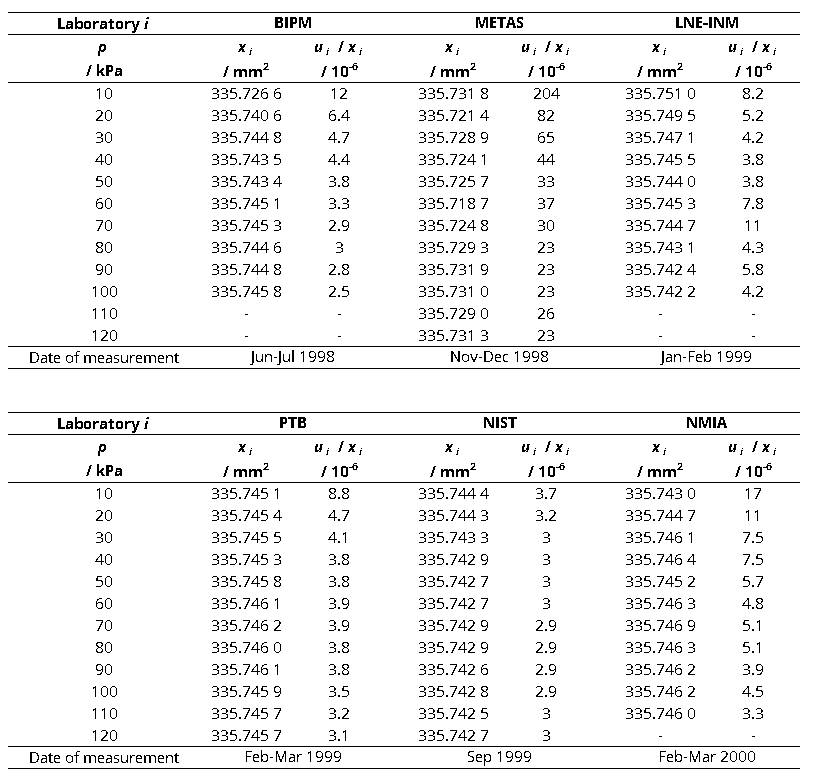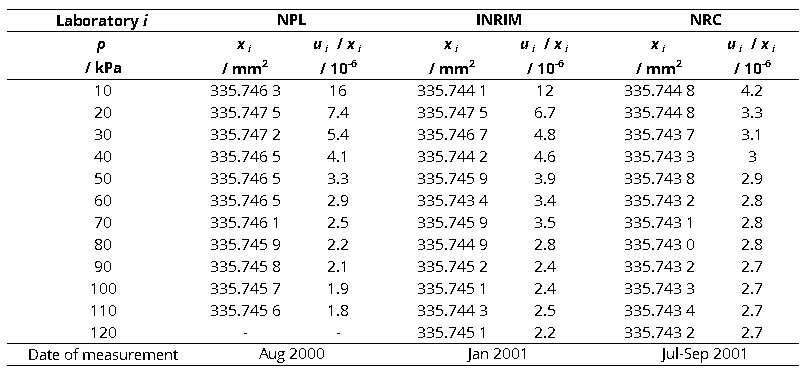CCM.P-K2 and EURAMET.M.P-K8

MEASURAND                     Effective area of a piston-cylinder assembly

NOMINAL VALUE              335.7 mm2

NOMINAL PRESSURE       20 kPa

xi   results obtained by laboratory i, Labi

ui   combined standard uncertainty of xiCCM.P-K2 and APMP.M.P-K9

MEASURAND                     Effective area of a piston-cylinder assembly

NOMINAL VALUE              335.7 mm2

NOMINAL PRESSURE       30 kPa

xi   results obtained by laboratory i, Labi

ui   combined standard uncertainty of xiCCM.P-K2

MEASURAND                     Effective area of a piston-cylinder assembly

NOMINAL VALUE              335.7 mm2

NOMINAL PRESSURE       40 kPa

xi   results obtained by laboratory i, Labi

ui   combined standard uncertainty of xiCCM.P-K2, EURAMET.M.P-K8 and APMP.M.P-K9

MEASURAND                     Effective area of a piston-cylinder assembly

NOMINAL VALUE              335.7 mm2

NOMINAL PRESSURE       50 kPa

xi   results obtained by laboratory i, Labi

ui   combined standard uncertainty of xiCCM.P-K2

MEASURAND                     Effective area of a piston-cylinder assembly

NOMINAL VALUE              335.7 mm2

NOMINAL PRESSURE       60 kPa

xi   results obtained by laboratory i, Labi

ui   combined standard uncertainty of xiCCM.P-K2, EURAMET.M.P-K8 and APMP.M.P-K9

MEASURAND                     Effective area of a piston-cylinder assembly

NOMINAL VALUE              335.7 mm2

NOMINAL PRESSURE       70 kPa

xi   results obtained by laboratory i, Labi

ui   combined standard uncertainty of xiCCM.P-K2

MEASURAND                     Effective area of a piston-cylinder assembly

NOMINAL VALUE              335.7 mm2

NOMINAL PRESSURE       80 kPa

xi   results obtained by laboratory i, Labi

ui   combined standard uncertainty of xiCCM.P-K2 and APMP.M.P-K9

MEASURAND                     Effective area of a piston-cylinder assembly

NOMINAL VALUE              335.7 mm2

NOMINAL PRESSURE       90 kPa

xi   results obtained by laboratory i, Labi

ui   combined standard uncertainty of xiCCM.P-K2, EURAMET.M.P-K8 and APMP.M.P-K9

MEASURAND                     Effective area of a piston-cylinder assembly

NOMINAL VALUE              335.7 mm2

NOMINAL PRESSURE       100 kPa

xi   results obtained by laboratory i, Labi

ui   combined standard uncertainty of xiCCM.P-K2 and APMP.M.P-K9

MEASURAND                     Effective area of a piston-cylinder assembly

NOMINAL VALUE              335.7 mm2

NOMINAL PRESSURE       110 kPa

xi   results obtained by laboratory i, Labi

ui   combined standard uncertainty of xiCCM.P-K2

MEASURAND                     Effective area of a piston-cylinder assembly

NOMINAL VALUE              335.7 mm2

NOMINAL PRESSURE       120 kPa

xi   results obtained by laboratory i, Labi

ui   combined standard uncertainty of xi